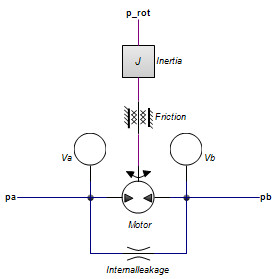﻿ 20-sim webhelp > Library > Iconic Diagrams > Hydraulics > Motors > displacementmotor-leakage

# displacementmotor-leakage

## Library

Iconic Diagrams\Hydraulics\Pumps

## Use

Domains: Continuous. Size: 1-D. Kind: Iconic Diagrams (Hydraulics).Description

This model describes an motor with internal leakage and an axial speed that is proportional to the input flow rate:

pa.phi = pb.phi = i * p_rot.omega;

i = D / (2*pi);

The actual flows at the inlet and outlet port may be slightly different because of the flow into the lumped volumes and the leakage flows. The leakage flows are modeled by laminar resistances. The torque is equal to:

p.T = i*(pa.p - pb.p);

If the port pressure is smaller than the vapour pressure (p < p_vapour), the flow is zero.

## Interface

 Ports Description pa pb p_rot inlet port (hydraulic) outlet port (hydraulic) axis (rotation) Causality preferred pressure out pa preferred pressure out pa preferred angular velocity out p_rot
 Parameters D J d_m Displacement per revolution [m3] Rotational inertia [kg.m2] Viscous (rotational) friction [N.m.s/rad] p_static Start pressure, acts as Coulomb friction, set to 2% of max pressure if unknown [Pa] G_int Internal laminar leakage conductance, set to 1e-13 if unknown [m3/s.Pa] V Dead volume of the pump at each port [m3]